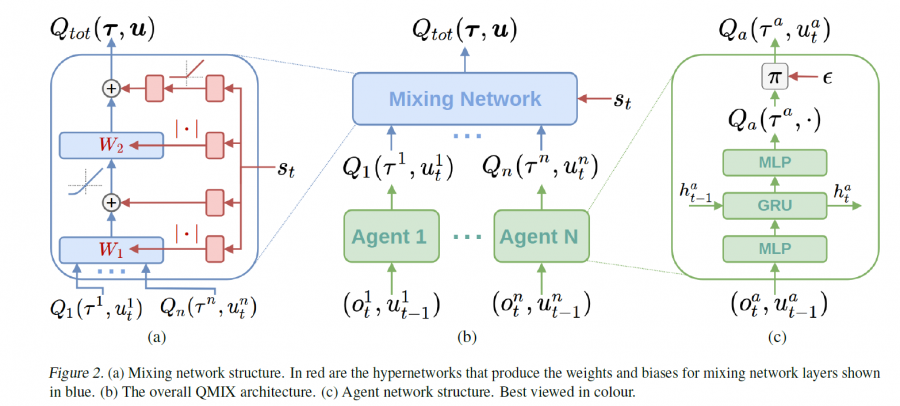# 多智能体强化学习算法

## QMIX：Monotonic Value Function Factorisation for Deep Multi-Agent Reinforcement Learning

Posted by MY on August 7, 2020

## 一、问题

IQL（independent Q-learning）是非常暴力的给每个智能体执行一个Q-learning算法，因为共享环境，并且环境随着每个智能体策略、状态发生改变，对每个智能体来说，环境是动态不稳定的，因此这个算法很难收敛。

Value-Decomposition Networks（VDN）的基本假设是，系统的联合$Q$函数可以近似为多个单智能体的$Q$函数的和。但VDN中联合函数的求和形式表现力有限，并不能涵盖更加复杂的组合情况，比如非线性组合。

## 二、解法

$\underset{\mathbf{u}}{\operatorname{argmax}} Q_{t o t}(\tau, \mathbf{u})=\left(\begin{array}{c}{\operatorname{argmax}_{u^{1}} Q_{1}\left(\tau^{1}, u^{1}\right)} \\ {\vdots} \\ {\operatorname{argmax}_{u^{n}} Q_{n}\left(\tau^{n}, u^{n}\right)}\end{array}\right)$

$\frac{\partial Q_{t o t}}{\partial Q_{a}} \geq 0, \forall a \in A$

QMIX模型由两部分组成，agent networks输出单智能体的$Q_{i}$函数，mix network 以$Q_{i}$作为输入，用于计算联合$Q$函数。为了满足单调的限制，mix network每一层的参数均由一个hypernetwork计算生成。hypernetworks以当前状态s作为输入，输出一个与mix network当前层形状相匹配的向量作为参数。hypernetworks隐藏层的激活函数是非负的（比如ReLU），这样得到的mix network每一层的参数也都是非负的，从而保证了单调的条件。同时使用hypernetwork相比于直接在网络中做约束会更灵活，没有bias限制。QMIX模型如下：QMIX的优化目标是最小化如下loss：

$\mathcal{L}(\theta)=\sum_{i=1}^{b}\left[\left(y_{i}^{t o t}-Q_{t o t}(\tau, \mathbf{u}, s ; \theta)\right)^{2}\right]$

## 三、实验内容## 五、优点

• QMIX提出了一种通用性更强的值函数分解方案，改进了VDN联合Q函数的形式
• 在mix network融合了部分全局信息提高了效果
• 实验环境难度有较大提升
• 使用hypernetwork是一种很巧妙的方式，值得借鉴。

##### Share